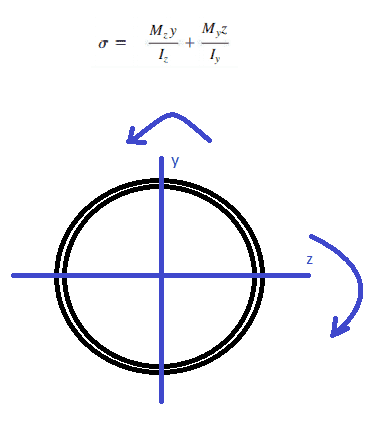# Question about max stress on circular cross section with two moments

• Engineering
• grotiare

#### grotiare

Homework Statement
This is more of a conceptual understanding. HW problems given do not deal with this specific scenario
Relevant Equations
σmax = (M*c)/I
I couldn't fit in the title, but this is with a hollow circular cross section

So currently I am trying to figure what occurs when two, perpendicular bending moments are applied to a hollow circular cross section (one about the z axis, and the other about y). I know that if I was dealing with a square cross-section, the stress caused by bending moment will be greatest at the corners and you simply add the max bending stress caused by each moment. But, for a circular cross section, I am unsure of the location of when the stresses are greatest. I attached a photo of what I am visualizing, thanks.

#### Attachments

•IMG_20210727_083823247.jpg
45.4 KB · Views: 75
•Delta2

For a linear-elastic, prismatic body with symmetric cross-section, the normal stress varies linearly with the distance from the neutral axis and the flexure formula is simply sigma=M*y/I... Just as in the case of a rectangular cross-section, the two resulting sets of normal stresses (from the applied moments) can simply be added together - or subtracted if, for instance, one produces compression and the other tension...Assuming Mz=My and Iz=Iy, the highest stress would occur where y+z is a maximum... Without thinking too much about it, I would expect this to occur at 45 degrees.

•grotiare and Lnewqban
Certain amount of those two moments will cancel each other and you will have one single equivalent moment acting on your circular section.

•grotiare
It is best to find the resultant moment and use the radius of the circle as the c value. The angle of the resultant moment can also be calculated using vector analysis.

•Dr.D and grotiare
Thanks guys for all the tips! I figured it out shortly after, but yes like what everyone above said I had to find the resultant moment of both, which can simply be found be treating the two moments as vectors and utilizing magnitude formula sqrt(x^2 +y^2) to get the magnitude, and then plugging that resultant magnitude into the stress from bending moment formula.

Last edited:
•PhanthomJay and Lnewqban